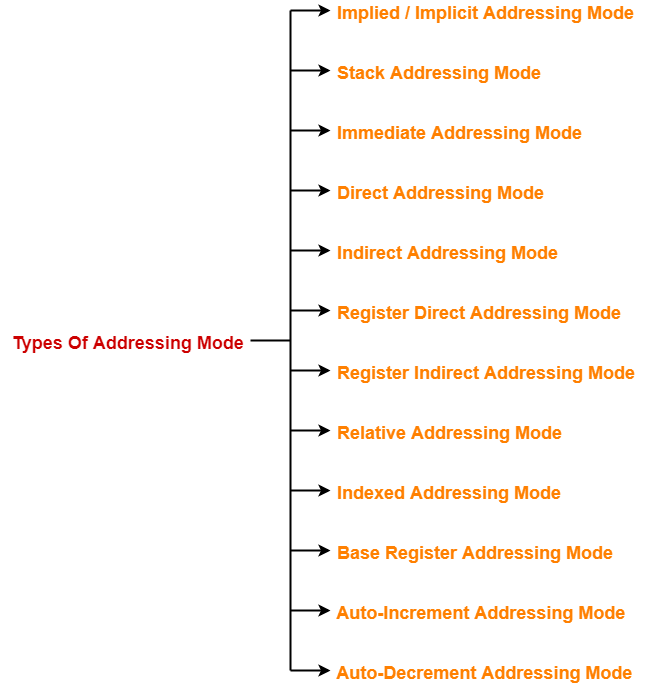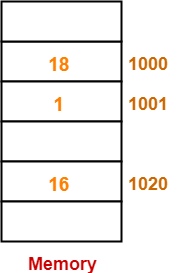# Addressing Modes | Practice Problems | COA

## Addressing Modes in Computer Architecture-

Before you go through this article, make sure that you have gone through the previous article on Addressing Modes.

We have discussed-

• Addressing modes are the different ways of specifying the location of an operand.
• Various kinds of addressing modes and their applications.## Problem-01:

The most appropriate matching for the following pairs is-

#### Column-2:

1. Loops

2. Pointers

3. Constants

1. X-3, Y-2, Z-1
2. X-1, Y-3, Z-2
3. X-2, Y-3, Z-1
4. X-3, Y-1, Z-2

## Solution-

Option (C) is correct.

## Problem-02:

1. The operand is inside the instruction
2. The address of the operand is inside the instruction
3. The register containing the address of the operand is specified inside the instruction
4. The location of the operand is implicit

## Solution-

Option (B) is correct.

## Problem-03:

Which of the following addressing modes are suitable for program relocation at run time?

1. 1 and 4
2. 1 and 2
3. 2 and 3
4. 1, 2 and 4

## Solution-

Option (C) is correct.

## Problem-04:

What is the most appropriate match for the items in the first column with the items in the second column-

#### Column-2:

1. Array implementation

2. Writing relocatable code

3. Passing array as parameter

1. X-3, Y-1, Z-2
2. X-2, Y-3, Z-1
3. X-3, Y-2, Z-1
4. X-1, Y-3, Z-2

## Solution-

Option (A) is correct.

## Problem-05:

Which of the following addressing modes permits relocation without any change whatsoever in the code?

## Solution-

Option (C) is correct.

## Problem-06:

Consider a three word machine instruction-

The first operand (destination) “A[R0]” uses indexed addressing mode with R0 as the index register. The second operand operand (source) “@B” uses indirect addressing mode. A and B are memory addresses residing at the second and the third words, respectively. The first word of the instruction specifies the opcode, the index register designation and the source and destination addressing modes. During execution of ADD instruction, the two operands are added and stored in the destination (first operand).

The number of memory cycles needed during the execution cycle of the instruction is-

1. 3
2. 4
3. 5
4. 6

## Solution-

For the first operand,

• It uses indexed addressing mode.
• Thus, one memory cycle will be needed to fetch the operand.

For the second operand,

• It uses indirect addressing mode.
• Thus, two memory cycles will be needed to fetch the operand.

After fetching the two operands,

• The operands will be added and result is stored back in the memory.
• Thus, one memory cycle will be needed to store the result.

Total number of memory cycles needed

=  1 + 2 + 1

= 4

Thus, Option (B) is correct.

## Problem-07:

Consider a hypothetical processor with an instruction of type LW R1, 20(R2), which during execution reads a 32-bit word from memory and stores it in a 32-bit register R1. The effective address of the memory location is obtained by the addition of a constant 20 and the contents of register R2. Which of the following best reflects the addressing mode implemented by this instruction for operand in memory?

## Solution-

Clearly, the instruction uses base indexed addressing mode.

Thus, Option (D) is correct.

## Problem-08:

The memory locations 1000, 1001 and 1020 have data values 18, 1 and 16 respectively before the following program is executed.

Which of the statements below is TRUE after the program is executed?

1. Memory location 1000 has value 20
2. Memory location 1020 has value 20
3. Memory location 1021 has value 20
4. Memory location 1001 has value 20

## Solution-

Before the execution of program, the memory is-Now, let us execute the program instructions one by one-

### Instruction-01: MOVI Rs, 1

• This instruction uses immediate addressing mode.
• The instruction is interpreted as Rs ← 1.
• Thus, value = 1 is moved to the register Rs.

• This instruction uses displacement addressing mode.
• The instruction is interpreted as Rd ← [1000 + [Rs]].
• Value of the operand = [1000 + [Rs]] = [1000 + 1] =  = 1.
• Thus, value = 1 is moved to the register Rd.

• This instruction uses immediate addressing mode.
• The instruction is interpreted as Rd ← [Rd] + 1000.
• Value of the operand = [Rd] + 1000 = 1 + 1000 = 1001.
• Thus, value = 1001 is moved to the register Rd.

### Instruction-04: STOREI 0(Rd), 20

• This instruction uses displacement addressing mode.
• The instruction is interpreted as 0 + [Rd] ← 20.
• Value of the destination address = 0 + [Rd] = 0 + 1001 = 1001.
• Thus, value = 20 is moved to the memory location 1001.

Thus,

• After the program execution is completed, memory location 1001 has value 20.
• Option (D) is correct.

## Problem-09:

Consider the following memory values and a one-address machine with an accumulator, what values do the following instructions load into accumulator?

• Word 20 contains 40
• Word 30 contains 50
• Word 40 contains 60
• Word 50 contains 70

Instructions are-

## Solution-

• This instruction uses immediate addressing mode.
• The instruction is interpreted as Accumulator ← 20.
• Thus, value 20 is loaded into the accumulator.

• This instruction uses direct addressing mode.
• The instruction is interpreted as Accumulator ← .
• It is given that word 20 contains 40.
• Thus, value 40 is loaded into the accumulator

• This instruction uses indirect addressing mode.
• The instruction is interpreted as Accumulator ← [].
• It is given that word 20 contains 40 and word 40 contains 60.
• Thus, value 60 is loaded into the accumulator.

• This instruction uses immediate addressing mode.
• The instruction is interpreted as Accumulator ← 30.
• Thus, value 30 is loaded into the accumulator.

• This instruction uses direct addressing mode.
• The instruction is interpreted as Accumulator ← .
• It is given that word 30 contains 50.
• Thus, value 50 is loaded into the accumulator

• This instruction uses indirect addressing mode.
• The instruction is interpreted as Accumulator ← [].
• It is given that word 30 contains 50 and word 50 contains 70.
• Thus, value 70 is loaded into the accumulator.

Next Article- System Bus in Computer Architecture

Get more notes and other study material of Computer Organization and Architecture.

Watch video lectures by visiting our YouTube channel LearnVidFun.

SummaryArticle Name
Addressing Modes | Practice Problems | COA
Description
Practice Problems based on Addressing Modes in computer architecture. Addressing modes specify the location of an operand. Various types of addressing modes exist in computer architecture.
Author
Publisher Name
Gate Vidyalay
Publisher Logo